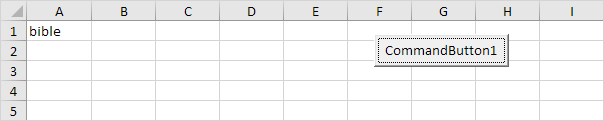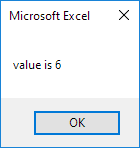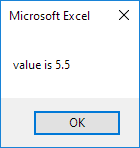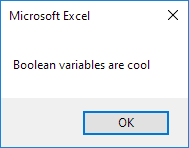# Variables

This chapter teaches you how to declare, initialize and display a variable in Excel VBA. Letting Excel VBA know you are using a variable is called declaring a variable. Initializing simply means assigning a beginning (initial) value to a variable.

Place a command button on your worksheet and add the code lines below. To execute the code lines, click the command button on the sheet.

### Integer

Integer variables are used to store whole numbers.

Dim x As Integer

x = 6

Range(“A1”).Value = x

Result:Explanation: the first code line declares a variable with name x of type Integer. Next, we initialize x with value 6. Finally, we write the value of x to cell A1.

### String

String variables are used to store text.

Code:

Dim book As String

book = “bible”

Range(“A1”).Value = book

Result:Explanation: the first code line declares a variable with name book of type String. Next, we initialize book with the text bible. Always use apostrophes to initialize String variables. Finally, we write the text of the variable book to cell A1.

### Double

A variable of type Double is more accurate than a variable of type Integer and can also store numbers after the comma.

Code:

Dim x As Integer

x = 5.5

MsgBox “value is ” & x

Result:But that is not the right value! We initialized the variable with value 5.5 and we get the value 6. What we need is a variable of type Double.

Code:

Dim x As Double

x = 5.5

MsgBox “value is ” & x

Result:Note: Long variables have even larger capacity. Always use variables of the right type. As a result, errors are easier to find and your code will run faster.

### Boolean

Use a Boolean variable to hold the value True or False.

Code:

Dim continue As Boolean

continue = True

If continue = True Then MsgBox “Boolean variables are cool”

Result:Explanation: the first code line declares a variable with name continue of type Boolean. Next, we initialize continue with the value True. Finally, we use the Boolean variable to only display a MsgBox if the variable holds the value True.